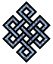#fail2ban bad ip database: ip 119.226.30.110

### | ip database | live view | stats | report | help | api key:

 ip: 119.226.30.110 hostname: 119.226.30.110 country:[IN] India first reported: 01.02.2017 07:09.52 GMT+0200 last reported: 24.11.2018 21:14.13 GMT+0200 time period: 661d 14h 04m 21s total reports: 10 reported by: 3 host(s) filter(s): ssh (6) ssh (4) tor exit node no badips.com db Lookup## port scan of '119.226.30.110':

[-hide]
```# Nmap 6.40 scan initiated Tue Nov 20 18:25:02 2018 as: /usr/bin/nmap -sU -sS -O 119.226.30.110
Nmap scan report for 119.226.30.110
Host is up (0.18s latency).
Not shown: 1000 open|filtered ports, 992 filtered ports
PORT     STATE  SERVICE
21/tcp   open   ftp
22/tcp   closed ssh
80/tcp   closed http
113/tcp  closed ident
443/tcp  closed https
3306/tcp open   mysql
8081/tcp open   blackice-icecap
8443/tcp open   https-alt
No exact OS matches for host (If you know what OS is running on it, see http://nmap.org/submit/ ).
TCP/IP fingerprint:
OS:SCAN(V=6.40%E=4%D=11/20%OT=21%CT=22%CU=%PV=N%G=Y%TM=5BF44444%P=x86_64-pc
OS:-linux-gnu)SEQ(SP=100%GCD=1%ISR=103%TI=Z%TS=A)OPS(O1=M5B4ST11NW7%O2=M5B4
OS:ST11NW7%O3=M5B4NNT11NW7%O4=M5B4ST11NW7%O5=M5B4ST11NW7%O6=M5B4ST11)WIN(W1
OS:=3890%W2=3890%W3=3890%W4=3890%W5=3890%W6=3890)ECN(R=Y%DF=Y%TG=40%W=3908%
OS:O=M5B4NNSNW7%CC=Y%Q=)T1(R=Y%DF=Y%TG=40%S=O%A=S+%F=AS%RD=0%Q=)T2(R=N)T3(R
OS:=N)T4(R=N)T5(R=Y%DF=Y%TG=40%W=0%S=Z%A=S+%F=AR%O=%RD=0%Q=)T6(R=N)T7(R=N)U
OS:1(R=N)IE(R=Y%DFI=N%TG=40%CD=S)

OS detection performed. Please report any incorrect results at http://nmap.org/submit/ .
# Nmap done at Tue Nov 20 18:28:36 2018 -- 1 IP address (1 host up) scanned in 215.46 seconds
```
```Σ = 34 | Δt = 0.0044839382171631s
```